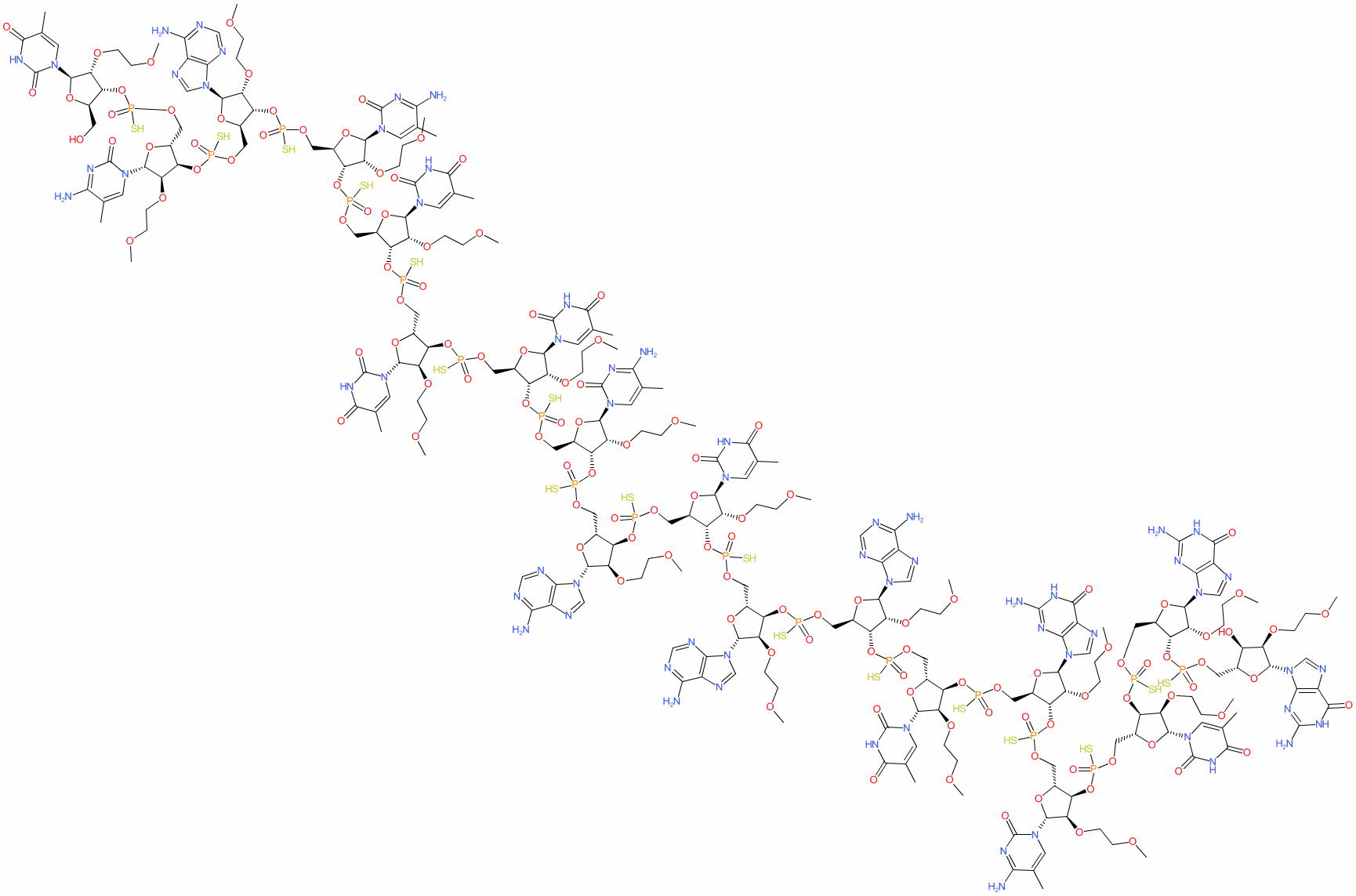#### GtoPdb Ligand ID: 9416

Synonyms: ASO-10-27 | Ionis-SMNrx | ISIS 396443 | ISIS-SMNRx | ISIS396443 | Spinraza®
nusinersen is an approved drug (FDA (2016), EMA (2017))
Compound class: Synthetic organic
Comment: Nusinersen (ISIS 396443) is an antisense oligo(ribo)nucleotide (ASO) which induces survival motor neuron (SMN) protein expression from SMN2 genes with the exon 7-skipping mutation .
Nusinersen was initially available for use as a designated orphan drug (by the US FDA and EMA), and was the first drug to be fully approved for the treatment of spinal muscular atrophy (SMA).

The sequence contains 2'-O-(2-methoxyethyl) (2'-MOE)-oligoribonucleotides to reduce nuclease degradation and enhance binding affinity towards the complementary RNA. The full sequence is [2'-O-(2-methoxyethyl)](3'-5')(P-thio)(mU-mC-A-mC-mU-mU-mU-mC-A-mU-A-A-mU-G-mC-mU-G-G) as detailed in the INN record for the agent. A full structural rendering using SMILES from Chemspider is available here (CSID:34983394). The sequence for nusinersen is claimed in patent WO2010148249A1 as SEQ ID NO: 1 .
2D StructurePhysico-chemical PropertiesHydrogen bond acceptors 161 Hydrogen bond donors 23 Rotatable bonds 176 Topological polar surface area 3144.17 Molecular weight 7122.28 XLogP -19.23 No. Lipinski's rules broken 3
SMILES / InChI / InChIKeyCanonical SMILES COCCOC1C(OP(=O)(OCC2OC(C(C2OP(=O)(OCC2OC(C(C2OP(=O)(OCC2OC(C(C2OP(=O)(OCC2OC(C(C2OP(=O)(OCC2OC(C(C2OP(=O)(OCC2OC(C(C2OP(=O)(OCC2OC(C(C2OP(=O)(OCC2OC(C(C2OP(=O)(OCC2OC(C(C2O)OCCOC)n2cnc3c2nc(N)[nH]c3=O)S)OCCOC)n2cnc3c2nc(N)[nH]c3=O)S)OCCOC)n2cc(C)c(=O)[nH]c2=O)S)OCCOC)n2cc(C)c(nc2=O)N)S)OCCOC)n2cnc3c2nc(N)[nH]c3=O)S)OCCOC)n2cc(C)c(=O)[nH]c2=O)S)OCCOC)n2cnc3c2ncnc3N)S)OCCOC)n2cnc3c2ncnc3N)S)OCCOC)n2cc(C)c(=O)[nH]c2=O)S)C(OC1n1cnc2c1ncnc2N)COP(=O)(OC1C(COP(=O)(OC2C(COP(=O)(OC3C(COP(=O)(OC4C(COP(=O)(OC5C(COP(=O)(OC6C(COP(=O)(OC7C(COP(=O)(OC8C(CO)OC(C8OCCOC)n8cc(C)c(=O)[nH]c8=O)S)OC(C7OCCOC)n7cc(C)c(nc7=O)N)S)OC(C6OCCOC)n6cnc7c6ncnc7N)S)OC(C5OCCOC)n5cc(C)c(nc5=O)N)S)OC(C4OCCOC)n4cc(C)c(=O)[nH]c4=O)S)OC(C3OCCOC)n3cc(C)c(=O)[nH]c3=O)S)OC(C2OCCOC)n2cc(C)c(=O)[nH]c2=O)S)OC(C1OCCOC)n1cc(C)c(nc1=O)N)S Isomeric SMILES COCCO[C@@H]1[C@H](OP(=O)(OC[C@H]2O[C@H]([C@@H]([C@@H]2OP(=O)(OC[C@H]2O[C@H]([C@@H]([C@@H]2OP(=O)(OC[C@H]2O[C@H]([C@@H]([C@@H]2OP(=O)(OC[C@H]2O[C@H]([C@@H]([C@@H]2OP(=O)(OC[C@H]2O[C@H]([C@@H]([C@@H]2OP(=O)(OC[C@H]2O[C@H]([C@@H]([C@@H]2OP(=O)(OC[C@H]2O[C@H]([C@@H]([C@@H]2OP(=O)(OC[C@H]2O[C@H]([C@@H]([C@@H]2OP(=O)(OC[C@H]2O[C@H]([C@@H]([C@@H]2O)OCCOC)n2cnc3c2nc(N)[nH]c3=O)S)OCCOC)n2cnc3c2nc(N)[nH]c3=O)S)OCCOC)n2cc(C)c(=O)[nH]c2=O)S)OCCOC)n2cc(C)c(nc2=O)N)S)OCCOC)n2cnc3c2nc(N)[nH]c3=O)S)OCCOC)n2cc(C)c(=O)[nH]c2=O)S)OCCOC)n2cnc3c2ncnc3N)S)OCCOC)n2cnc3c2ncnc3N)S)OCCOC)n2cc(C)c(=O)[nH]c2=O)S)[C@H](O[C@H]1n1cnc2c1ncnc2N)COP(=O)(O[C@@H]1[C@@H](COP(=O)(O[C@@H]2[C@@H](COP(=O)(O[C@@H]3[C@@H](COP(=O)(O[C@@H]4[C@@H](COP(=O)(O[C@@H]5[C@@H](COP(=O)(O[C@@H]6[C@@H](COP(=O)(O[C@@H]7[C@@H](COP(=O)(O[C@@H]8[C@@H](CO)O[C@H]([C@@H]8OCCOC)n8cc(C)c(=O)[nH]c8=O)S)O[C@H]([C@@H]7OCCOC)n7cc(C)c(nc7=O)N)S)O[C@H]([C@@H]6OCCOC)n6cnc7c6ncnc7N)S)O[C@H]([C@@H]5OCCOC)n5cc(C)c(nc5=O)N)S)O[C@H]([C@@H]4OCCOC)n4cc(C)c(=O)[nH]c4=O)S)O[C@H]([C@@H]3OCCOC)n3cc(C)c(=O)[nH]c3=O)S)O[C@H]([C@@H]2OCCOC)n2cc(C)c(=O)[nH]c2=O)S)O[C@H]([C@@H]1OCCOC)n1cc(C)c(nc1=O)N)S InChI Key WWFDJIVIDXJAQR-FFWSQMGZSA-N
 ClassificationCompound class Synthetic organic Approved drug? Yes (FDA (2016), EMA (2017))
 International Nonproprietary NamesINN number INN 10003 nusinersen
 SynonymsASO-10-27 | Ionis-SMNrx | ISIS 396443 | ISIS-SMNRx | ISIS396443 | Spinraza®
 Database LinksCAS Registry No. 1258984-36-9 (source: WHO INN record) GtoPdb PubChem SID 328083518 PubChem CID 124037382 Search Google for chemical match using the InChIKey WWFDJIVIDXJAQR-FFWSQMGZSA-N Search Google for chemicals with the same backbone WWFDJIVIDXJAQR Search PubMed clinical trials nusinersen Search PubMed titles nusinersen Search PubMed titles/abstracts nusinersen UniChem Compound Search for chemical match using the InChIKey WWFDJIVIDXJAQR-FFWSQMGZSA-N UniChem Connectivity Search for chemical match using the InChIKey WWFDJIVIDXJAQR-FFWSQMGZSA-N